# SAT Math Multiple Choice Question 335: Answer and Explanation

### Test Information

Question: 335

5. Which of the following systems of inequalities has no solution?

• A.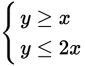• B.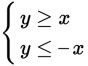• C.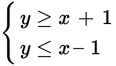• D.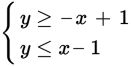Explanation:

C

Difficulty: Medium

Category: Heart of Algebra / Inequalities

Strategic Advice: You don't need to use algebra to answer this question, and you also don't need to graph each system. Instead, think about how the graphs would look. The only time a system of inequalities has no solution is when it consists of two parallel lines shaded in opposite directions.

Getting to the Answer: All the inequalities are written in slope-intercept form, so look for parallel lines (two lines that have the same slope but different y-intercepts). The slopes in A are different (m = 1 and m = 2), so eliminate this choice. The same is true for B (m = 1 and m = -1) and D (m = -1 and m = 1). This means (C) must be correct (m = 1 and m = 1, b = 1 and b = -1). The graph of the system is shown here: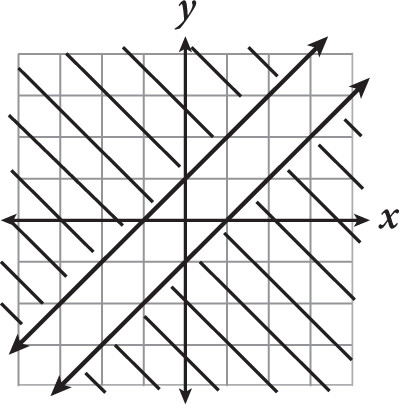Because the shading never overlaps, the system has no solution.